2019/04/10 10:10

# 1. 我们为什么需要态势预测

• 定量预测：利用原始数据和信息，借助数学模型和方法，分析数据前后之间的关系，得出其未来的发展变化规律，进而达到预测目的，主要方法有
• 时间序列
• 人工神经网络
• 灰色理论
• 回归分析
• 定性预测：主要依靠个人的经验积累和能力，利用有限的原始数据进行推理、判断和估测，主要方法有
• 专家评估法
• 类推法
• 判断分析法
• 市场调查法

• 首先获取历史态势数据序列
• 运用技术方法处理和变换数据序列
• 然后利用数学模型，发现和识别安全态势数据序列之间的关系和规律，建立包含时间变量、态势变量的方程关系式
• 通过求解方程得到随时间变化的态势函数

# 2. 态势预测是否是伪科学？什么场景下可以进行态势预测？

## 0x1：《周易》所谓的见微知著

• 网络安全攻防的主体是黑客，是量子态思想的人，对不可预测的黑客来说，为何发起、何时发起、针对谁发起攻击都是未知且不可预测的。如果攻击者不可预测，那么如何能预测受害者何时遭受到攻击呢
• 不仅仅是发起网络攻击的黑客，就连服务器的合法管理员，其本身的行为模式也是难以预测的，这就导致服务器处于正常状态时，其运行时系统行为数据序列往往也呈现出无规律的波动，这就导致了时序模型无法有效建模，更不用提未来预测了

《周易·说卦传》里写道：“数往者顺，知来者逆，是故易逆数也。

《周易》认为未来是可以通过“逆数”而预知的，“逆数”与“顺数”正相反，

• “顺数”是自多而寡，自上而下
• “逆数”却是自少生多，自下积上

“下”就是事之始，“上”便是事之成”。邵雍在《皇极经世》里说：“知古亦未必为古，今亦未必为今，皆自我而观之也。

《周易》里预知未来的方法非常复杂，我们只简单的介绍一个容易理解的方法——“知几”。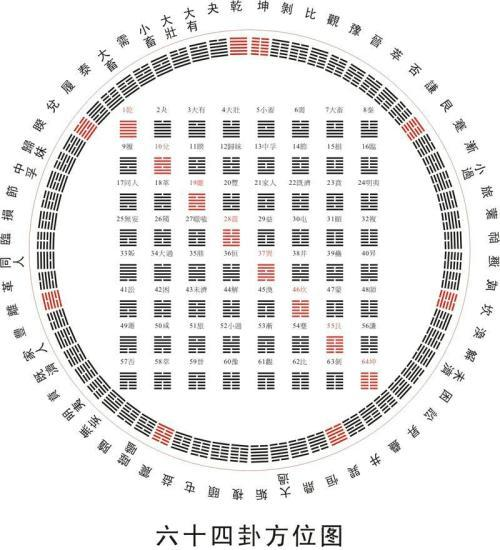• 当纣王开始用象牙做筷子时，箕子就察觉到奢侈之风即将到来，酒池肉林、鹿台琼室即将出现，百姓也会因此而离心王室，因此发出长叹；
• 当晋武帝平定吴国之后，务在骄奢，不复留心治政。何曾就察觉到晋朝已时日无多，将来必有内乱；
• 明朝人万二读到朱元璋的诗，里面说：“百僚未起朕先起，百僚已睡朕未睡。不如江南富足翁，日高五丈犹披被。”他立即看破了端倪，马上把家资付托予人，自己泛舟而逃，两年后许多江南大族果然被朝廷抄没。“知几”在于发现事物变化的端倪，然后采取行动来趋吉避凶。但更为关键的是，我们应该用什么办法来发现这个端倪呢？

• 日食和月食就是我们所见到的“”，
• 而造成这些“象”的内在本质原因、内在的“”就是天体间的运行规律，
• 至于那个“某时某刻某地”则是最终的“”。

• 霞与气象为我们所见之“象”，
• 霞反映了空中云层的变化，故能表现天气，这是内在的“理”，
• 而“朝”与“晚”就是“数”；

## 0x2：经济学视角下的未来态势预测

• 潜在增速决定了经济运行的均衡趋势，
• 经济周期决定了经济运行的波动态势。

• 宏观调控的核心是分析经济形势并实施反周期操作，通过采用财政政策、货币政策等进行削峰填谷式的操作，熨平波动，促进经济平稳运行。反思大萧条诞生了凯恩斯主义，伯南克在研究大萧条时声称找到了避免大萧条的办法，2008年以后美联储采取QE进行货币再膨胀，开启了美国历史上最长的经济复苏。
• 资产配置的核心是研究有效解决资源稀缺问题，美林投资时钟是资产配置领域的经典方法，通过对经济增长和通胀两个指标的分析，将经济周期分为衰退、复苏、过热、滞胀四个阶段，并依次推荐债券、股票、大宗商品、现金。美国、中国等的历史数据验证了投资时钟的有效性。

• 短波的农业周期（又称蛛网周期）揭示的是农业对价格的生产反馈周期，1年左右；
• 中短波的库存周期（又称基钦周期）揭示的是工商业部门的存货调整周期，3年左右；
• 中长波的设备投资周期（又称朱格拉周期）揭示的是产业在生产设备和基础设施的循环投资活动，10年左右；
• 长波的建筑周期（又称库兹涅茨周期）主要是住房建设活动导致的，30年左右；
• 超长波的创新周期（又称康德拉耶夫周期）是由创新活动的集聚发生及退潮所致，60年左右。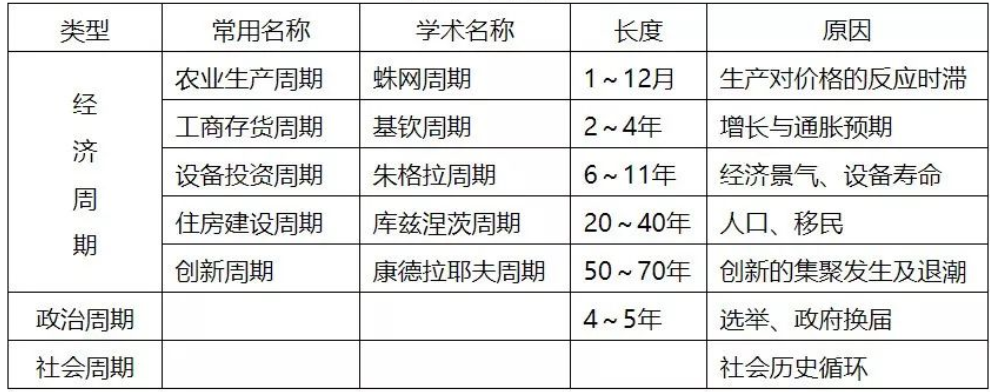• 初始的驱动因素包括太阳活动、过度投资、有效需求不足、创新、政策冲击等，
• 放大机制包括乘数加速数、抵押品信贷、货币加速器（即商业银行的顺周期行为）、情绪等。

## 0x3：国家宏观经济中GDP中的未来态势预测

GDP预测不仅是宏观经济学研究中的重要课题，很多非经济学专业人士对此也颇感兴趣。目前学界所使用的GDP预测方法或预测模型可以根据输入数据的不同，粗略地分为两大类：

• 历史数据拟合模型，它们的共同特点是：只需要输入GDP历史数据，通过对已知数据的拟合来尝试归纳出未来的发展趋势。具体方法则有
• 移动平均法
• 指数平滑法
• 插值法
• 时间序列模型
• ARIMA模型
• 马尔可夫链模型等等
• 多维输入模型：对于历史数据拟合模型来说，不管这类方法得出的预测结果本身有多么准确，都无法回答大家最关心的问题：我们应该为了改善经济/经济的可持续发展做些什么？当前的经济发展趋势将在何时迎来拐点？为了更好地辅助决策，我们往往希望得到一个更加有指导意义的GDP预测模型，这样的模型除了以往的GDP数据，还需要其他的输入，而且能够评估这些输入对预测结果的影响。
• 通过多维度的输入数据来预测GDP，这类模型往往带有鲜明的“自定义特色”，不同的作者对于哪些经济社会指标与GDP的关系更紧密往往有自己的判断，而这些判断在实际的数据验证过程中有些可能得到支持，而有些则可能被否定。

• 明天的太阳几点升起
• 下一个月潮将于几点到来
• 哈雷彗星的回归准确时间

## 0x4：传播学视角下的未来态势预测

• 为什么支付宝的晒账单、微信的晒历史会迅速刷屏？
• 为什么芙蓉姐姐、凤姐、庞麦郎会迅速走红？
• 为什么大家都说杜蕾斯的社会化营销做得最好？它的营销方案的哪些特点让其能够屡次引发病毒式传播？
• 你最近向身边的人强力推荐过什么？背后有哪些原因？

• social currency：我们分享让我们看起来棒棒哒东西；
• trigger：我们分享正好在脑袋里想起来的东西；
• emotion：我们分享让我们情绪高度唤起的东西；
• public：想让别人分享，必须先让更多人看见；
• practical value：我们分享有价值、有用处的东西；
• stories：如何让情节帮助进行信息传播;

• 对于政府而言，可以感知当前的舆情和疫情态势，从而采取措施来控制网络舆情和大规模流行病的爆发；
• 对于网络电商而言，可以采取个性化推荐策略来营销产品；
• 对于金融系统而言，可以预警早期金融风险，进而采取措施防止全球性金融危机爆发。

• WNCRY勒索病毒在传播的同时，关于其解决方案和预防措施的信息也在快速扩散，在极大程度上降低了经济损失；
• 由于HIV患者的免疫系统受到了损坏，他们更容易被其他传染病性疾病感染；
• 2019Ecov冠状病毒在传播时，相关国家政府机构也在同时采取强力的管控措施，同时民众对疫情的认识和重视程度也在逐步增加，这导致实际的疫情传播结果是一个复杂因素的共演化结果；

• 共演化生物传播（Coevolution of biological contagions）
• 共演化社会传播（Coevolution of social contagions）
• 意识—流行病传播（Coevolution of awareness diffusion and epidemic spreading）
• 资源—流行病传播（Coevolution of resource diffusion and epidemic spreading）四大类

• SIS
• SIR
• CP模型

• 异质平均场
• 淬火平均场
• 动态信息传递
• 点对近似
• 边渗流理论等

### 3. 意识—流行病传播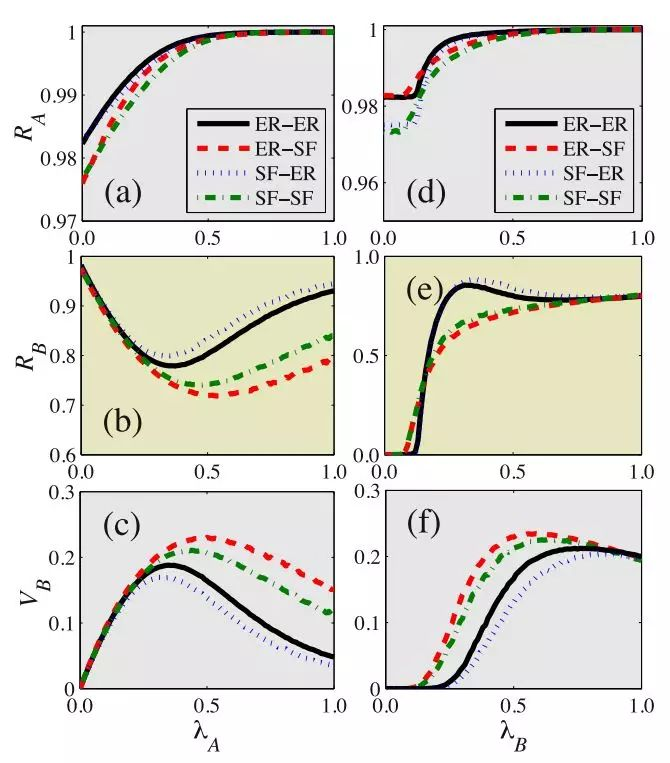### 4. 资源—流行病传播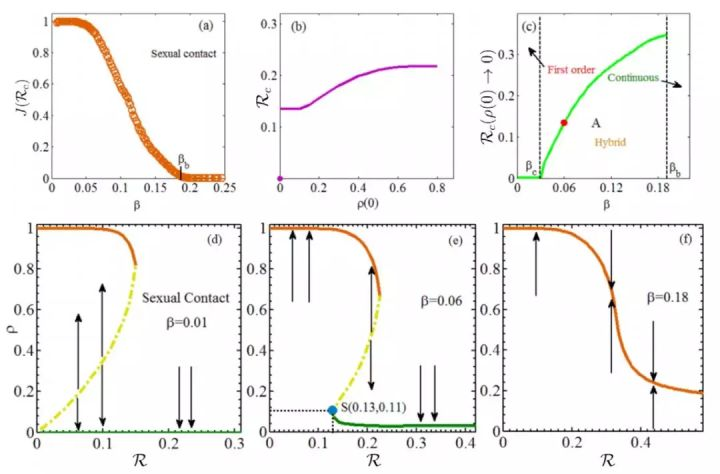http://www.360doc.com/showweb/0/0/894564652.aspx
http://finance.sina.com.cn/stock/stockzmt/2019-04-06/doc-ihvhiqax0497038.shtml
https://user.guancha.cn/main/content?id=30156
https://www.sec-un.org/%E5%AE%89%E5%85%A8%E7%9A%84%E8%BF%9B%E5%8C%96%E8%AE%BA%EF%BC%88%E4%BA%8C%EF%BC%89%EF%BC%9A%E6%9D%A5%E8%AF%B4%E8%AF%B4%E6%80%81%E5%8A%BF%E6%84%9F%E7%9F%A5/
http://blog.sciencenet.cn/blog-40841-1084903.html
https://www.zhihu.com/question/20162816
https://zhuanlan.zhihu.com/p/88916343

# 3. 基于灰色理论进行态势预测

## 0x2：灰色系统简介

• 系统因素不完全明确
• 因素关系不完全清楚
• 系统结构不完全知道
• 系统作用原理不完全明了

• 以灰色朦胧集为基础的理论体系
• 灰色朦胧集、灰色代数系统、灰色矩阵等是灰色理论的基础
• 以灰色关联空间为依托的分析体系：灰色系统分析主要包括：
• 灰色关联分析
• 灰色聚类
• 灰色统计评估
• 以灰色序列生成为基础的方法体系：灰色序列生成通过序列算子来实现，灰色序列算子主要包括：
• 缓冲算子
• 均值生成算子
• 以灰色模型未核心的模型体系：灰色模型则按照五个步骤进行模型构建，
• 通过灰色生成或序列算子的作用弱化随机性来挖掘潜在规律，
• 经过灰色差分方程与灰色微分方程之间的互换来实现“利用离散的数据序列建立连续的动态微分方程”的新飞跃
• 以系统分析、评估、建模、预测、决策、控制、优化为主体的技术体系
• 灰色预测是基于GM模型做出的定量预测，可分为多种类型
• 灰色决策包括灰色关联决策、灰色统计、灰色层次决策、灰色局势决策等
• 灰色控制包括本证性灰色系统的控制问题和以灰色系统方法为基础构成的控制，如灰色关联控制和GM(1，1)预测控制等
• 灰色优化技术包括灰色线性规划、灰色非线性规划、灰色整数规划和灰色动态规划等。

## 0x3：灰色理论建立依据

• 灰色理论将随机量当做在一定范围内变化的灰色量，将随机过程当做在一定范围、一定时区内变化的灰色过程
• 灰色系统将无规律的历史数据序列经累加后，使其变为具有指数增长规律的上升形状数列，由于一阶微分方程解的形式是指数增长形式，所以可对生成后数列建立微分方程模型。所以灰色模型实际上是生成数列所建模型
• 灰色理论通过灰色的不同生成方式、数据的不同取舍、不同级别的残差GM模型来调整、修正、提高精度
• 对高阶系统建模，灰色理论是通过GM(1，N)模型群解决的。GM(1，N)模型群也是一阶微分方程组的灰色模型
• GM模型所得数据必须经过逆生长，即累减生成还原后才能应用

• 用原始数据组成原始序列(0)，经累加生成法生成序列(1)，它可以弱化原始数据的随机性，使其呈现出较为明显的特征规律。
• 对生成变换后的序列(1) 建立微分方程型的模型即GM模型。GM(1，1) 模型表示1阶的、1个变量的微分方程模型。
• 用GM(1，1) 模型进行预测，精度较高的仅仅是原点数据(0)(n) 以后的1到2个数据，即预测时刻越远预测的意义越弱。这是因为任何一个灰色系统在发展过程中，随着时间的推移，将会不断地有一些随即扰动和驱动因素进入系统，使系统的发展相继地受其影响。
• 为了解决1阶GM(1，1)表征和描述能力不足的问题，我们可以基于1阶的GM模型继续衍生为高阶GM模型，即GM(1，1) 模型群。在GM(1，1)模型群中，新陈代谢模型是最理想的模型。新陈代谢GM(1，1)模型的基本思想为，越接近的数据，对未来的影响越大，同一个输入信息，随着时间的推移，其对趋势的影响会逐渐减小。也就是说，在不断补充新信息的同时，去掉意义不大的老信息，这样的建模序列更能动态地反映系统最新的特征，这实际上是一种动态预测模型。

## 0x4：灰色模型的应用场景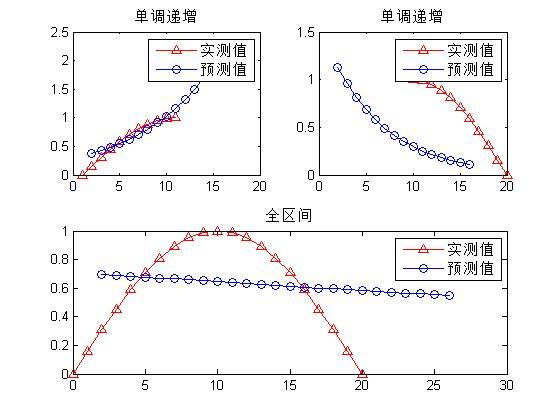• 灰色预测对于单调变化的序列预测精度较高，但是对波动变化明显的序列而言，灰色预测的误差相对比较大。
• 究其原因，灰色预测模型通过AGO累加生成序列，在这个过程中会将不规则变动视为干扰，在累加运算中会过滤掉一部分变动，而且由累加生成灰指数律定理可知，当序列足够大时，存在级比为0.5的指数律，这就决定了灰色预测对单调变化预测具有很强的惯性，使得波动变化趋势不敏感。clc
clear all
% 本程序主要用来计算根据灰色理论建立的模型的预测值。
% 应用的数学模型是 GM(1,1)。
% 原始数据的处理方法是一次累加法。
x=[0:1:10];
x1=[10:1:20];
x2=[0:1:20];
y=sin(pi*x/20);
n=length(y);
yy=ones(n,1);
yy(1)=y(1);
for i=2:n
yy(i)=yy(i-1)+y(i);
end
B=ones(n-1,2);
for i=1:(n-1)
B(i,1)=-(yy(i)+yy(i+1))/2;
B(i,2)=1;
end
BT=B';
for j=1:n-1
YN(j)=y(j+1);
end
YN=YN';
A=inv(BT*B)*BT*YN;
a=A(1);
u=A(2);
t=u/a;
t_test=5;  %需要预测个数
i=1:t_test+n;
yys(i+1)=(y(1)-t).*exp(-a.*i)+t;
yys(1)=y(1);
for j=n+t_test:-1:2
ys(j)=yys(j)-yys(j-1);
end
x=1:n;
xs=2:n+t_test;
yn=ys(2:n+t_test);
det=0;
for i=2:n
det=det+abs(yn(i)-y(i));
end
det=det/(n-1);

subplot(2,2,1),plot(x,y,'^r-',xs,yn,'b-o'),title('单调递增' ),legend('实测值','预测值');
disp(['百分绝对误差为：',num2str(det),'%']);
disp(['预测值为： ',num2str(ys(n+1:n+t_test))]);

%递减
y1=sin(pi*x1/20);
n1=length(y1);
yy1=ones(n1,1);
yy1(1)=y1(1);
for i=2:n1
yy1(i)=yy1(i-1)+y1(i);
end
B1=ones(n1-1,2);
for i=1:(n1-1)
B1(i,1)=-(yy1(i)+yy1(i+1))/2;
B1(i,2)=1;
end
BT1=B1';
for j=1:n1-1
YN1(j)=y1(j+1);
end
YN1=YN1';
A1=inv(BT1*B1)*BT1*YN1;
a1=A1(1);
u1=A1(2);
t1=u1/a1;
t_test1=5;  %需要预测个数
i=1:t_test1+n1;
yys1(i+1)=(y1(1)-t1).*exp(-a1.*i)+t1;
yys1(1)=y1(1);
for j=n1+t_test1:-1:2
ys1(j)=yys1(j)-yys1(j-1);
end
x21=1:n1;
xs1=2:n1+t_test1;
yn1=ys1(2:n1+t_test1);
det1=0;
for i=2:n1
det1=det1+abs(yn1(i)-y1(i));
end
det1=det1/(n1-1);

subplot(2,2,2),plot(x1,y1,'^r-',xs1,yn1,'b-o'),title('单调递增' ),legend('实测值','预测值');
disp(['百分绝对误差为：',num2str(det1),'%']);
disp(['预测值为： ',num2str(ys1(n1+1:n1+t_test1))]);

%整个区间
y2=sin(pi*x2/20);
n2=length(y2);
yy2=ones(n2,1);
yy2(1)=y2(1);
for i=2:n2
yy2(i)=yy2(i-1)+y2(i);
end
B2=ones(n2-1,2);
for i=1:(n2-1)
B2(i,1)=-(yy2(i)+yy2(i+1))/2;
B2(i,2)=1;
end
BT2=B2';
for j=1:n2-1
YN2(j)=y2(j+1);
end
YN2=YN2';
A2=inv(BT2*B2)*BT2*YN2;
a2=A2(1);
u2=A2(2);
t2=u2/a2;
t_test2=5;  %需要预测个数
i=1:t_test2+n2;
yys2(i+1)=(y2(1)-t2).*exp(-a2.*i)+t2;
yys2(1)=y2(1);
for j=n2+t_test2:-1:2
ys2(j)=yys2(j)-yys2(j-1);
end
x22=1:n2;
xs2=2:n2+t_test2;
yn2=ys2(2:n2+t_test2);
det2=0;
for i=2:n2
det2=det2+abs(yn2(i)-y2(i));
end
det2=det2/(n2-1);

subplot(2,1,2),plot(x2,y2,'^r-',xs2,yn2,'b-o'),title('全区间' ),legend('实测值','预测值');
disp(['百分绝对误差为：',num2str(det2),'%']);
disp(['预测值为： ',num2str(ys2(n2+1:n2+t_test2))]);
View Code

## 0x5：灰色模型的公理化定义

### 1. GM(1，1)模型

x(1) = (x(1)(1)，x(1)(2)，x(1)(3)，....，x(1)(n))，其中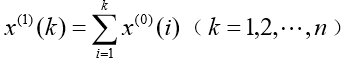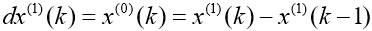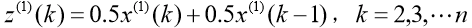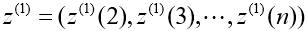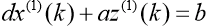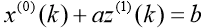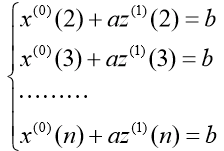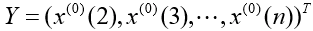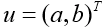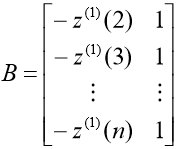，称 Y 为数据向量，B 为数据矩阵，u 为参数向量，则 GM(1，1)模型可以表示为矩阵方程 Y = Bu。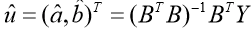### 2. GM(1，N)模型

GM(1，N)的定义原理上和GM(1，1)是类似的。
GM(1，1)表示的模型是是 1 阶方程，且只含 1 个变量的灰色模型。而 GM(1，N) 即表示模型是 1 阶方程，但包含有 N 个变量的灰色模型。

## 0x6：灰色预测模型

### 1. 灰色预测的场景分类

• 时间序列预测：即用观察到的反映预测对象特征的时间序列来构造灰色预测模型，预测未来某一时刻的特征量，或者达到某一特征量的时间
• 畸变预测：即通过灰色模型预测异常值出现的时刻，预测异常值什么时候出现在特定时区内，或者指通过灰色模型预测灾变值发生在一年内某个特定的时区或季节的灾变预测
• 拓朴预测：通过原始数据作曲线，在曲线上按照特定值寻找该定值发生的所有时点，并以该定值为框架构成时点数列，然后建立模型来预测该定值所发生的时点
• 系统预测：通过对系统行为特征指标建立一组相互关联的灰色预测模型，预测系统中众多变量间相互协调关系的变化

• 利用 GM 模型对系统行为特征的发展变化规律进行估计预测，同时也可以对行为特征的异常情况发生的时刻进行估计计算，以及对在特定时区内发生事件的未来时间分布情况做出研究等等。这些工作实质上是将“随机过程”当作“灰色过程”，“随机变量”当作“灰变量”，并主要以灰色系统理论中的 GM(1,1)模型来进行处理。

### 2. 灰色预测模型数理化描述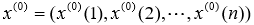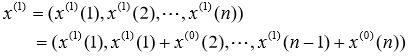，其中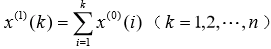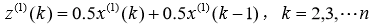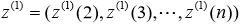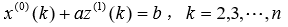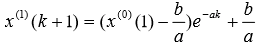，k=0，1，....，n-1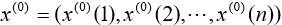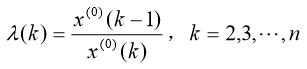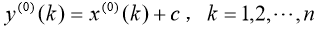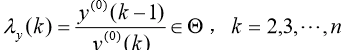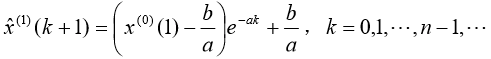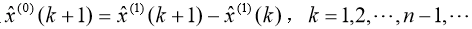• 残差检验
• 级比偏差值检验

（1）残差检验：零残差为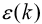，计算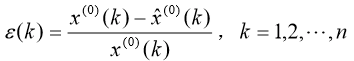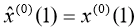（2）级比偏差值检验：首先由参考数据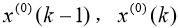计算出级比 λ(k)，再用发展系数 a 求出相应的级比偏差：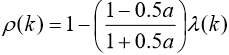### 3. 灰色预测建模过程

1. 把原始数据加工成生成数
2. 对残差（模型计算值与实际值之差）修订后，建立差分微分方程模型
3. 进行基于关联度收敛的分析
4. GM模型所得数据进行逆生成还原
5. 采用”五步建模“法，建立一种差分微分方程模型，即GM(1，1)预测模型。所谓五步建模包括
1. 系统定性分析
2. 因素分析
3. 初步量化
4. 动态量化
5. 优化

• 单序列灰色预测模型
• 区间灰数预测模型

• 累加生成
• 累减生成
• 均值生成
• 级比生成

• GM(1，1)模型
• DGM(1，1)模型
• GM(1，N)模型
• 灰色Verhulst模型

## 0x7：基于灰色模型进行预测的示例

### 1. 某地区未来降雨量灾变预测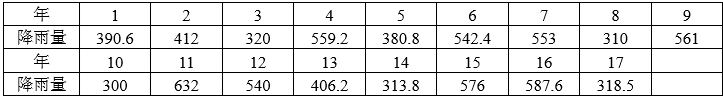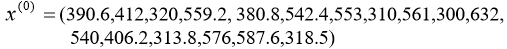# -*- coding: utf-8 -*-

import numpy as np
import matplotlib.pyplot as plt

y = np.array([390.6, 412, 320, 559.2, 380.8, 542.4, 553, 310, 561, 300, 632, 540, 406.2, 313.8, 576, 587.6, 318.5])
x = np.linspace(0, len(y), len(y), endpoint=True)

plt.figure(1)
# plt.plot(x,c)
plt.plot(x, y, color="blue", linewidth=3.0, linestyle="-", label="raint data", alpha=0.9)  # 颜色 线宽 类型 标签 透明度

# 坐标轴的操作
# 坐标轴的位置
ax = plt.gca()  # 引入坐标轴
ax.spines["right"].set_color("none")
ax.spines["top"].set_color("none")
ax.spines["left"].set_position(("data", 0))
ax.spines["bottom"].set_position(("data", 0))

# 坐标轴的刻度显示位置
ax.xaxis.set_ticks_position("bottom")
ax.yaxis.set_ticks_position("left")

# 设置坐标的显示范围
plt.yticks(np.linspace(-1, 1, 5, endpoint=True))  # 设置坐标的显示范围
# 设置刻度数字大小和边框
for lable in ax.get_xticklabels() + ax.get_yticklabels():
lable.set_fontsize(16)  # 刻度大小
lable.set_bbox(dict(facecolor="white", edgecolor="None", alpha=0.2))  # 刻度下面的小边框

# 图例
plt.legend(loc="upper left")

# 网格线
plt.grid()

plt.show()
View Code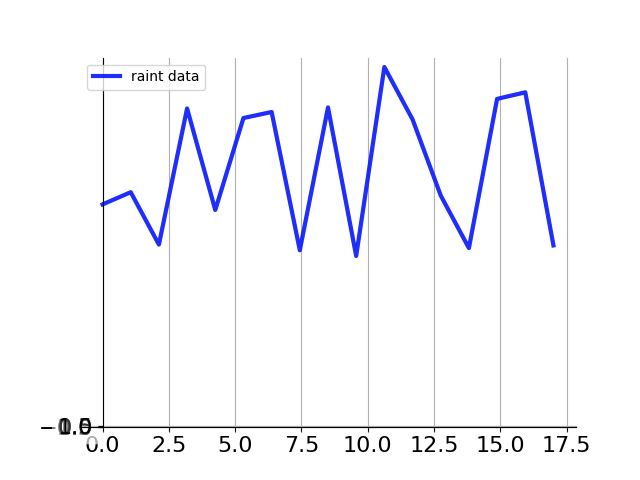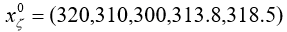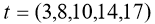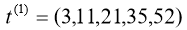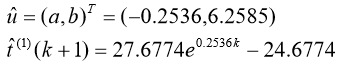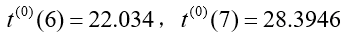• 22.034 - 17 / 22.034 = 0.2284
• 28.3946 - 17 / 28.3946 = 0.4012

GM模型针对包含周期性和连续趋势性的数列，具备较好的信息捕获与预测能力。GM(1，1)模型适用于具有较强指数规律的序列，只能描述单调的变化过程。

### 2. 利用灰色模型GM(1,1)预测长江污水排放量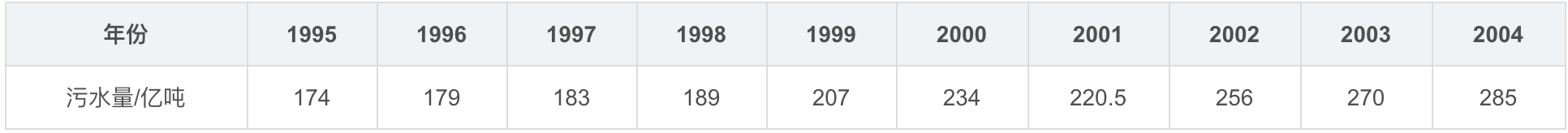1995-2004年的长江污水排放量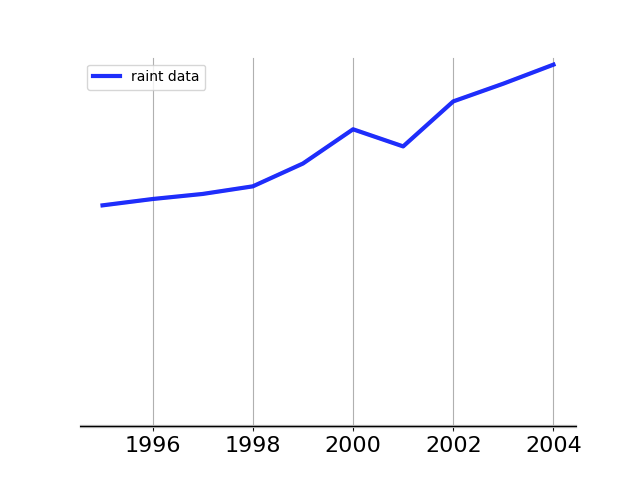%建立符号变量a(发展系数)和b(灰作用量)
syms a b;
c = [a b]';

%原始数列 A
A = [174, 179, 183, 189, 207, 234, 220.5, 256, 270, 285];
n = length(A);

%对原始数列 A 做累加得到数列 B
B = cumsum(A);

%对数列 B 做紧邻均值生成
for i = 2:n
C(i) = (B(i) + B(i - 1))/2;
end
C(1) = [];

%构造数据矩阵
B = [-C;ones(1,n-1)];
Y = A; Y(1) = []; Y = Y';

%使用最小二乘法计算参数 a(发展系数)和b(灰作用量)
c = inv(B*B')*B*Y;
c = c';
a = c(1); b = c(2);

%预测后续数据
F = []; F(1) = A(1);
for i = 2:(n+10)
F(i) = (A(1)-b/a)/exp(a*(i-1))+ b/a;
end

%对数列 F 累减还原,得到预测出的数据
G = []; G(1) = A(1);
for i = 2:(n+10)
G(i) = F(i) - F(i-1); %得到预测出来的数据
end

disp('预测数据为：');
G

%模型检验

H = G(1:10);
%计算残差序列
epsilon = A - H;

%法一：相对残差Q检验
%计算相对误差序列
delta = abs(epsilon./A);
%计算相对误差Q
disp('相对残差Q检验：')
Q = mean(delta)

%法二：方差比C检验
disp('方差比C检验：')
C = std(epsilon, 1)/std(A, 1)

%法三：小误差概率P检验
S1 = std(A, 1);
tmp = find(abs(epsilon - mean(epsilon))< 0.6745 * S1);
disp('小误差概率P检验：')
P = length(tmp)/n

%绘制曲线图
t1 = 1995:2004;
t2 = 1995:2014;

plot(t1, A,'ro'); hold on;
plot(t2, G, 'g-');
xlabel('年份'); ylabel('污水量/亿吨');
legend('实际污水排放量','预测污水排放量');
title('长江污水排放量增长曲线');
grid on;
View Code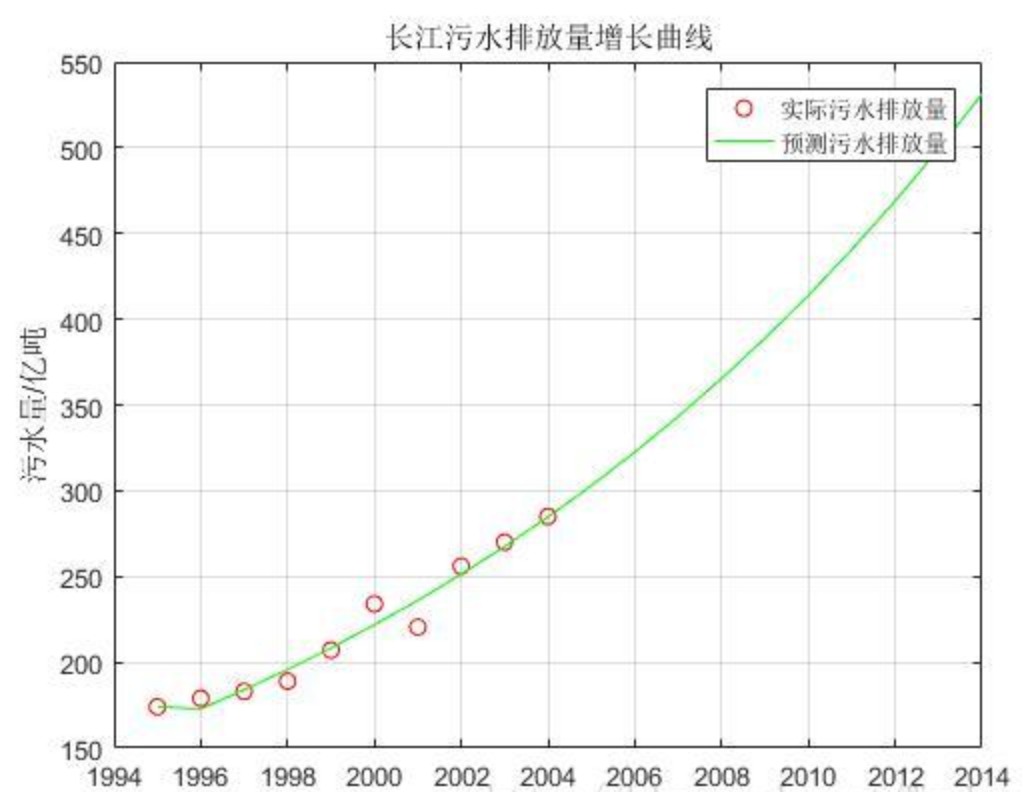### 3. 利用灰色Verhulst模型(即Logistic模型)预测大肠杆菌菌种繁殖速度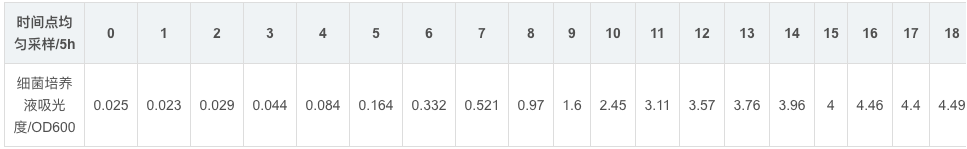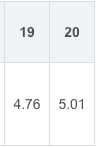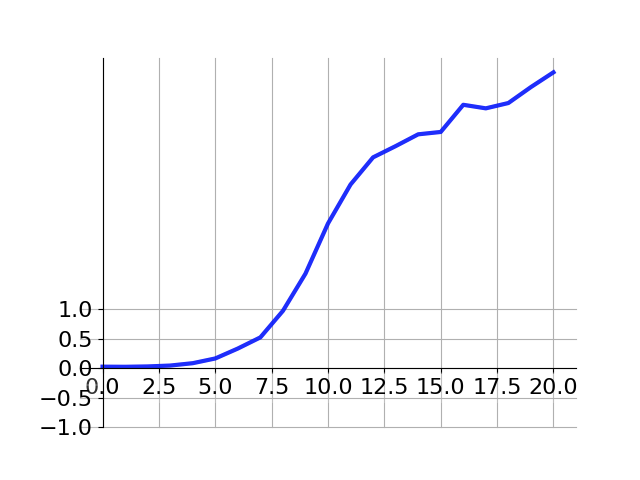%建立符号变量a(发展系数)和b(灰作用量)
syms a b;
c = [a b]';

%原始数列 A
A = [0.025, 0.023, 0.029, 0.044, 0.084, 0.164, 0.332, 0.521, 0.97, 1.6, 2.45, 3.11, 3.57, 3.76, 3.96, 4, 4.46, 4.4, 4.49, 4.76, 5.01];
n = length(A);

%对原始数列 A 做累减得到数列 B
for i = 2:n
H(i) = A(i) - A(i - 1);
end
H(1) = [];

%对原始数列 A 做紧邻均值生成
for i = 2:n
C(i) = (A(i) + A(i-1))/2;
end
C(1) = [];

%构造数据矩阵
D = [-C; C.^2];
Y = H; Y = Y';

%使用最小二乘法计算参数 a(发展系数)和b(灰作用量)
c = inv(D*D')*D*Y;
c = c';
a = c(1); b = c(2);

%得到预测出的数据
F = []; F(1) = A(1);
for i = 2:(n+n)
F(i) = (a*A(1))/(b*A(1)+(a - b*A(1))*exp(a*(i-1)));
end

disp('预测数据为：');
F

%绘制曲线图
t1 = 0:n-1;
t2 = 0:2*n-1;
plot(t1, A, 'ro'); hold on;
plot(t2, F);
xlabel('时间点均匀采样/5h'); ylabel('细菌培养液吸光度/OD600');
legend('实际数量','预测数量');
title('大肠杆菌培养S形增长曲线');
grid on;
View Code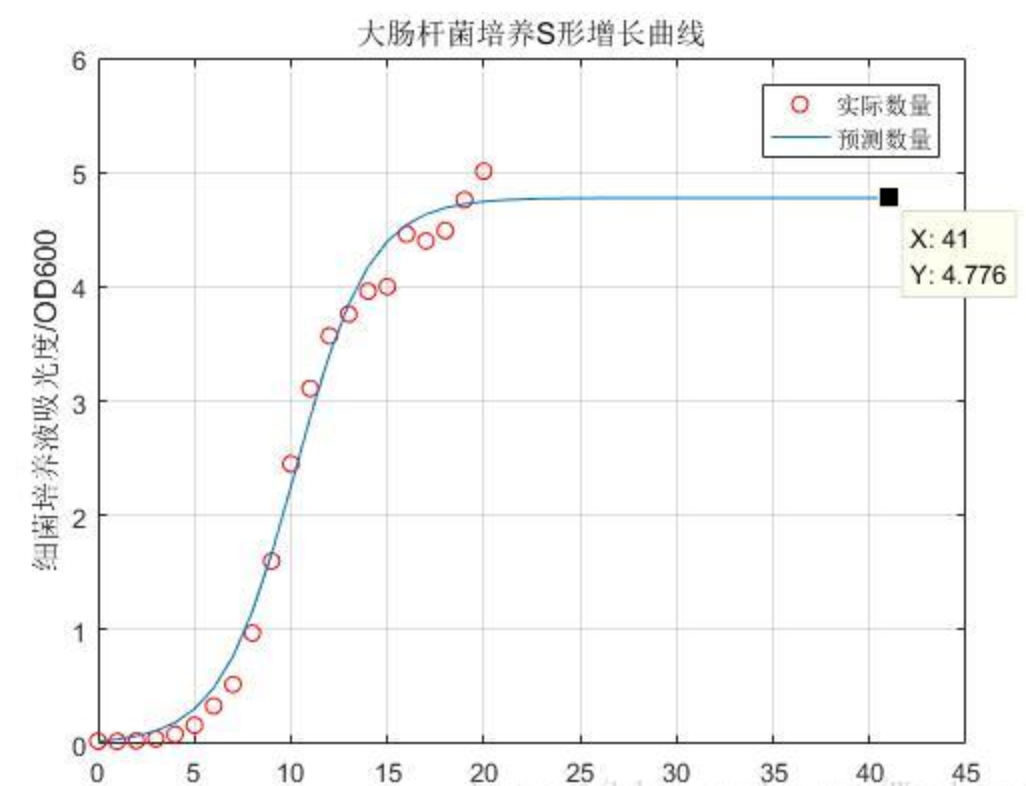• 趋势信息
• 周期性信息
• 统计规律性的随机信息

https://zhuanlan.zhihu.com/p/40811400
https://wiki.mbalib.com/wiki/%E7%81%B0%E8%89%B2%E7%B3%BB%E7%BB%9F%E7%90%86%E8%AE%BA
https://www.cnblogs.com/ECJTUACM-873284962/p/6721913.html
https://cloud.tencent.com/developer/article/1086549
https://blog.csdn.net/jiede1/article/details/54381257
https://blog.csdn.net/WuchangI/article/details/79214882 

# 4. 基于时间序列模型进行态势预测

## 0x2：时间序列分析的基本特征

• 一是时间序列分析根据过去的变化趋势预测未来的发展，它的前提是假定事物的过去延续到未来。时间序列分析正是根据客观事物发展的连续规律性，运用过去的历史数据，通过统计分析以进一步推测未来的发展趋势。事物的过去会延续到未来这个假设前提包含两层含义：
• 不会发生突然的跳跃变化，即以相对小的的步伐前进
• 过去和当前的现象可能表明现在和将来活动的发展变化趋向
• 二是时间序列数据变动存在着规律性与不规律性。时间序列中的每个观察值大小是影响变化的各种不同因素在同一时刻发生作用的综合结果，从这些影响因素发生作用的大小和方向变化的时间特性来看，这些因素造成的时间序列数据的变动分为四种类型：
• 趋势性：某个变量随着时间进展或自变量变化，呈现一种比较缓慢而长期的持续上升、下降、停留的同性质变动趋向，但变动幅度可能不相等
• 周期性：由于外部影响随着自然季节的交替出现高峰与低谷的规律
• 随机性：个别为随机变动因素，整体呈现统计规律
• 综合性：实际情况为几种变动的叠加或组合

https://www.cnblogs.com/LittleHann/p/11177458.html 

# 5. 基于回归分析模型进行态势预测

## 0x2：回归分析的定义和思路

• 函数关系：客观事物之间的确定性关系，也可以称为因果关系。可以用一个确定的数学表达式来反映
• 相关关系：反映事物之间的非严格、不确定的线性依存关系，难以用函数精确表达。对于这类关系，我们常用回归分析和相关分析来进行测度
• 相关分析是研究两个或两个以上随机变量之间线性依存关系的紧密程度的方法，通常用相关系数表示
• 回归分析则是研究某一随机变量（因变量）与其他一个或几个普通变量（自变量）之间数量变动关系的方法，它有着明确的自变量和因变量，这是与相关分析显著不同的地方

• 从一组样本数据出发，确定变量之间的数学关系式，对这些关系式的可信程度进行各种统计检验，并从影响某一特定变量的诸多变量中找出影响显著的变量子集。
• 然后利用所求的关系式，根据一个或几个变量的取值来预测或控制另一个特定变量的取值，并给出这种预测或者控制的精确程度

• 磁盘落盘了一个web文件，而该web文件通过行为判断确定其是一个webshell，而且这个web文件所在的路径又在外部可访问web目录下
• 从命令行审计中，发现有一个进程向注册表自启动目录下添加了一个可疑的自启动项，根据安全人员的先验经验，这个行为有99%的置信度代表了服务器被入侵了

## 0x3：回归模型的种类

• 根据回归分析涉及的自变量的多少，回归模型可以分为一元回归模型多元回归模型。在实际工程应用中 ，一元回归是非常少见的，大部分是高维的多元回归模型
• 根据模型中自变量和因变量之间的关系是否为线性，可以分为线性回归模型非线性回归模型，
• 如果回归分析中自变量和因变量的关系可以用一条直线近似表示，或者说两类变量数据分布大体上呈现直线趋势，这种回归模型称为线性回归模型
• 如果自变量和因变量的关系无法用一条直线近似表示，则为非线性回归模型，也称为曲线回归模型

## 0x4：回归分析预测的步骤

• 根据预测目标确定自变量和因变量：明确预测的具体目标，也就确定了因变量，如预测具体目标是攻击行为趋势，那么攻击行为趋势就是因变量。通过数据采集和态势提取，寻找与预测目标相关的影响因素，即自变量，并从中选出主要的影响因素
• 建立回归预测模型：根据自变量和因变量的历史统计数据进行计算，在此基础上建立回归分析方程，也就是回归分析预测模型
• 建立相关分析：回归分析是对具有因果关系的影响因素（自变量）和预测对象（因变量）所进行的数理统计分析处理，只有当自变量与因变量确实存在某种关系时，建立的回归方程才有意义。因此，作为自变量的因素与作为因变量的预测对象是否有关、相关程度如何，以及判断这种相关程度的把握性多大，就成为进行回归分析必须要解决的问题。进行相关分析，一般需要求出相关关系，以相关关系的大小来判断自变量和因变量的相关程度
• 校验回归分析预测模型，计算预测误差：回归分析预测模型是否可用于实际预测，取决于对回归分析预测模型的检验和对预测误差的计算。回归方程只有通过各种检验，且预测误差较小，才能将回归方程作为预测模型进行预测
• 计算并确定预测值：最后，利用回归分析预测模型计算预测值，并对预测值进行综合分析以确定最后的预测值

## 0x5：回归分析预测方法

### 2. 逻辑回归

• 病毒在传播初期，由于政府和民众重视程度不够，传染数字指数增长，也即J型曲线，增长不受抑制，呈爆炸式的。比如一个人可以传染三个人，三个人传染九个人，九个人传染27个人，不停的倍增。
• 在疫情出现之后，全国各地对确诊或疑似患者进行隔离，阻止了部分病毒的传播，因此传染的速度会逐渐受到压制，总体上上看，Logistic增长模型适用于nconv疫情传播前中期的趋势。

#### 1）logistic增长函数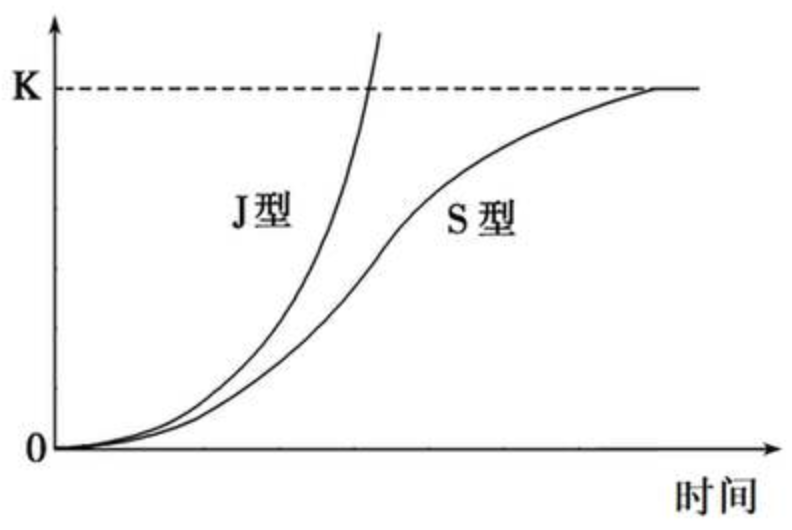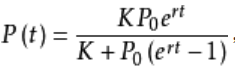• K为环境容量，即增长到最后，P(t)能达到的极限。
• P0为初始容量，就是t=0时刻的数量。
• r为增长速率，r越大则增长越快，越快逼近K值，r越小增长越慢，越慢逼近K值。
def logistic_increase_function(t,K,P0,r):
# t:time   t0:initial time    P0:initial_value    K:capacity  r:increase_rate
exp_value=np.exp(r*(t-t0))
return (K*exp_value*P0)/(K+(exp_value-1)*P0)

#### 2）历史数据准备

date    num
2020/1/10    41
2020/1/11    41
2020/1/12    41
2020/1/13    41
2020/1/14    41
2020/1/15    41
2020/1/16    45
2020/1/17    62
2020/1/18    121
2020/1/19    198
2020/1/20    291
2020/1/21    440
2020/1/22    571
2020/1/23    830
2020/1/24    1287
2020/1/25    1975
2020/1/26    2744
2020/1/27    4515
2020/1/28    5974
2020/1/29    7711
2020/1/30    9692
2020/1/31    11791
2020/2/1    14380
2020/2/2    17205
2020/2/3    20438
2020/2/4    24324
2020/2/5    28018
2020/2/6    31161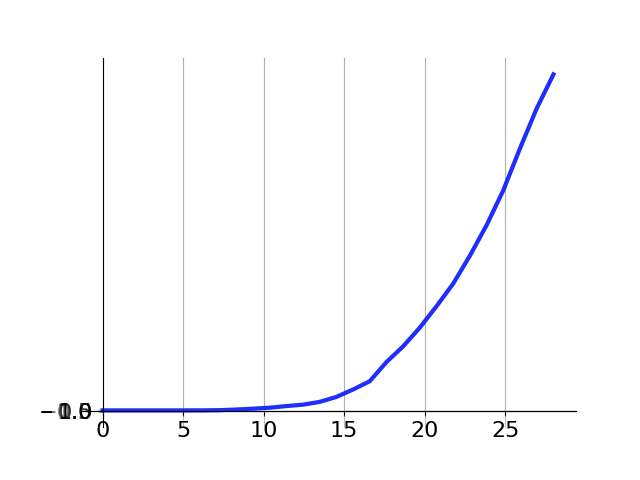#### 3）用最小二乘法进行序列拟合#!/usr/bin/python
# -*- coding: UTF-8 -*-

import numpy as np
import matplotlib.pyplot as plt
import math
import pandas as pd

import numpy as np
import matplotlib.pyplot as plt
from scipy.optimize import curve_fit

def logistic_increase_function(t, K, P0, r):
t0 = 10
r = 0.5
# t:time   t0:initial time    P0:initial_value    K:capacity  r:increase_rate
exp_value = np.exp(r * (t - t0))
return (K * exp_value * P0) / (K + (exp_value - 1) * P0)

#  日期及感染人数
t = [
10, 11, 12, 13, 14, 15, 16, 17, 18, 19, 20, 21, 22, 23,
24, 25, 26, 27, 28, 29, 30, 31,
32, 33, 34, 35, 36, 37
]
t = np.array(t)
P = [
41, 41, 41, 41, 41, 41, 45, 62, 121, 198, 291, 440, 571, 830, 1287,
1975, 2744, 4515, 5974, 7711, 9692, 11791,
14380, 17205, 20438, 24324, 28018, 31161
]
P = np.array(P)

# 用最小二乘法估计拟合
popt, pcov = curve_fit(logistic_increase_function, t, P)
# 获取popt里面是拟合系数
print("K:capacity  P0:initial_value   r:increase_rate   t:time")
print(popt)

# 拟合后预测的P值
P_predict = logistic_increase_function(t, popt, popt, popt)
# 未来预测
future = [
10, 11, 12, 13, 14, 15, 16, 17, 18, 19, 20, 21, 22, 23,
24, 25, 26, 27, 28, 29, 30, 31,
32, 33, 34, 35, 36, 37,
38, 39, 40, 41, 42, 43, 44, 45, 46, 47, 48, 49, 50, 51,
52, 53, 54, 55, 56, 57, 58, 59, 60, 61, 62, 63, 64, 65,
66, 67, 68, 69, 70, 71, 72, 73, 74, 75, 76, 77, 78, 79,
80, 81, 82, 83, 84, 85, 86
]
future = np.array(future)
future_predict = logistic_increase_function(future, popt, popt, popt)
# 近期情况预测
tomorrow = [
38, 39, 40, 41, 42, 43, 44, 45, 46, 47, 48, 49, 50, 51
]
tomorrow = np.array(tomorrow)
tomorrow_predict = logistic_increase_function(tomorrow, popt, popt, popt)

# 绘图
plot1 = plt.plot(t, P, 's', label="confimed infected people number")
plot2 = plt.plot(t, P_predict, 'r', label='predict infected people number')
plot3 = plt.plot(tomorrow, tomorrow_predict, 's', label='predict infected people number')
plt.xlabel('time')
plt.ylabel('confimed infected people number')

plt.legend(loc=0)  # 指定legend的位置右下角

print(logistic_increase_function(np.array(28), popt, popt, popt))
print(logistic_increase_function(np.array(29), popt, popt, popt))
plt.show()

print("Program done!")
View Code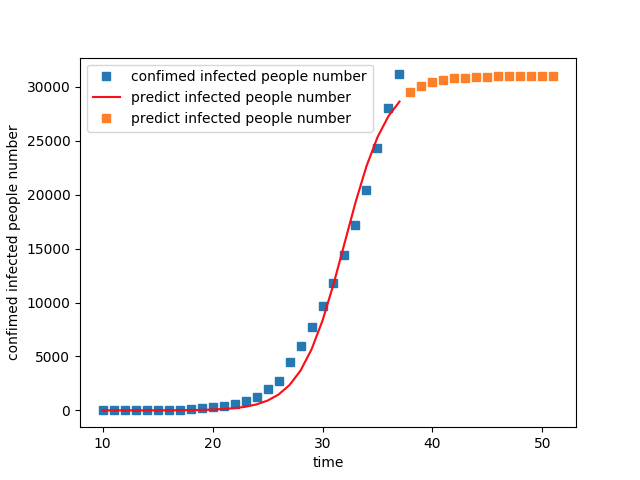#!/usr/bin/python
# -*- coding: UTF-8 -*-

import numpy as np
import matplotlib.pyplot as plt
import math
import pandas as pd

import numpy as np
import matplotlib.pyplot as plt
from scipy.optimize import curve_fit

def logistic_increase_function(t, K, P0, r):
t0 = 10
r = 0.2
# t:time   t0:initial time    P0:initial_value    K:capacity  r:increase_rate
exp_value = np.exp(r * (t - t0))
return (K * exp_value * P0) / (K + (exp_value - 1) * P0)

#  日期及感染人数
t = [
10, 11, 12, 13, 14, 15, 16, 17, 18, 19, 20, 21, 22, 23,
24, 25, 26, 27, 28, 29, 30, 31,
32, 33, 34, 35, 36, 37, 38, 39, 40, 41,
42, 43, 44, 45, 46, 47, 48, 49, 50, 51
]
t = np.array(t)
P = [
41, 41, 41, 41, 41, 41, 45, 62, 121, 198, 291, 440, 571, 830, 1287, 1975, 2744, 4515, 5974, 7711, 9692, 11791,
14380, 17205, 20438, 24324, 28018, 31161, 34546, 37198, 40171, 42638, 44653, 59804, 63851, 66492, 68500, 70548,
72436, 74185, 75002, 75891
]
P = np.array(P)

# 用最小二乘法估计拟合
popt, pcov = curve_fit(logistic_increase_function, t, P)
# 获取popt里面是拟合系数
print("K:capacity  P0:initial_value   r:increase_rate   t:time")
print(popt)

# 拟合后预测的P值
P_predict = logistic_increase_function(t, popt, popt, popt)
# 未来预测
future = [
10, 11, 12, 13, 14, 15, 16, 17, 18, 19, 20, 21, 22, 23,
24, 25, 26, 27, 28, 29, 30, 31,
32, 33, 34, 35, 36, 37, 38, 39, 40, 41,
42, 43, 44, 45, 46, 47, 48, 49, 50, 51,
52, 53, 54, 55, 56, 57, 58, 59, 60, 61, 62, 63, 64, 65,
66, 67, 68, 69, 70, 71, 72, 73, 74, 75, 76, 77, 78, 79,
80, 81, 82, 83, 84, 85, 86, 87, 88, 89, 90, 91, 92, 93,
94, 95, 96, 97, 98, 99, 100
]
future = np.array(future)
future_predict = logistic_increase_function(future, popt, popt, popt)
# 近期情况预测
tomorrow = [
52, 53, 54, 55, 56, 57, 58, 59, 60, 61, 62, 63, 64, 65,
66, 67, 68, 69, 70, 71, 72, 73, 74, 75, 76, 77, 78, 79
]
tomorrow = np.array(tomorrow)
tomorrow_predict = logistic_increase_function(tomorrow, popt, popt, popt)

# 绘图
plot1 = plt.plot(t, P, 's', label="confimed infected people number")
plot2 = plt.plot(t, P_predict, 'r', label='predict infected people number')
plot3 = plt.plot(tomorrow, tomorrow_predict, 's', label='predict infected people number')
plt.xlabel('time')
plt.ylabel('confimed infected people number')

plt.legend(loc=0)  # 指定legend的位置右下角

print(logistic_increase_function(np.array(28), popt, popt, popt))
print(logistic_increase_function(np.array(29), popt, popt, popt))
plt.show()

print("Program done!")
View Code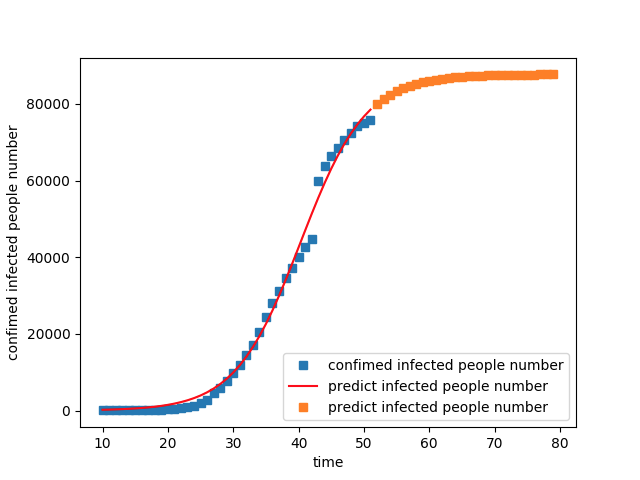### 7. ElasticNet回归

https://blog.csdn.net/weixin_36474809/article/details/104101055 

# 6. 基于动力学模型进行态势预测

• 系统动力学模型
• 吸附动力学模型
• 机器人动力学模型
• 微生物动力学模型
• 发酵动力学模型
• 药代动力学模型
• 传染病动力学模型
• 药物动力学模型
• 反应动力学模型
• 人工湿地动力学模型
• 神经动力学模型
• 互联网动力学模型
• 微扰量子色动力学模型
• 生物动力学模型
• 等等。

## 0x2：传染病动力学模型

### 1. SI模型

• 易感状态S（Susceptible）：一个个体在感染前是处于易感状态的，即该个体有可能被邻居个体感染
• 感染状态I（Infected）：一个感染上某种病毒的个体就称为是处于感染状态。，即该个体还会以一定概率感染其邻居个体
• 移除状态R（Remove，Recovered)：也称为免疫状态或恢复状态，当一个个体经历过一个完整的感染周期后，该个体就不再被感染，因此模型中就可以不再考虑该个体

SI传播模型是最简单的疾病传播模型，模型中的所有个体都只可能处于两个状态中的一个，即易感(S)状态或感染(I)状态。

S(t) + I(t) 恒等于 N

ds/dt = -β*S(t)I(t)/N

ds/dt = βS(t)*I(t)/N#!/usr/bin/python
# -*- coding: utf-8 -*-

import scipy.integrate as spi
import numpy as np
import pylab as pl

beta=1.4247
"""the likelihood that the disease will be transmitted from an infected to a susceptible
individual in a unit time is β"""
gamma=0
#gamma is the recovery rate and in SI model, gamma equals zero
I0=1e-6
#I0 is the initial fraction of infected individuals
ND=70
#ND is the total time step
TS=1.0
INPUT = (1.0-I0, I0)

def diff_eqs(INP,t):
'''The main set of equations'''
Y=np.zeros((2))
V = INP
Y = - beta * V * V + gamma * V
Y = beta * V * V - gamma * V
return Y   # For odeint

t_start = 0.0; t_end = ND; t_inc = TS
t_range = np.arange(t_start, t_end+t_inc, t_inc)
RES = spi.odeint(diff_eqs,INPUT,t_range)
"""RES is the result of fraction of susceptibles and infectious individuals at each time step respectively"""
print(RES)

#Ploting
pl.plot(RES[:,0], '-bs', label='Susceptibles')
pl.plot(RES[:,1], '-ro', label='Infectious')
pl.legend(loc=0)
pl.title('SI epidemic without births or deaths')
pl.xlabel('Time')
pl.ylabel('Susceptibles and Infectious')
pl.savefig('2.5-SI-high.png', dpi=900) # This does increase the resolution.
pl.show()
View Code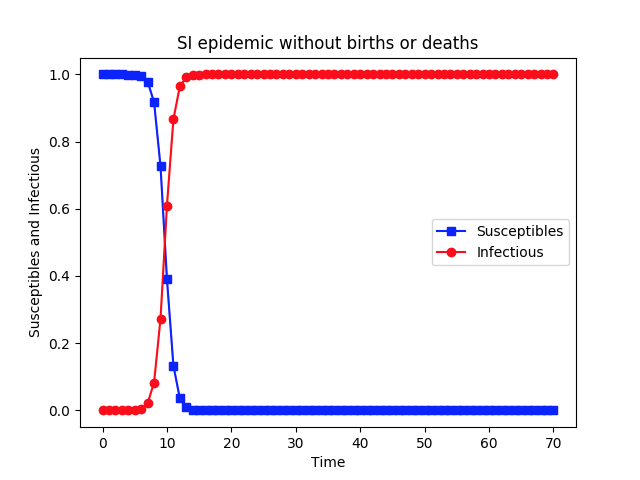### 2. SIS: 普通流感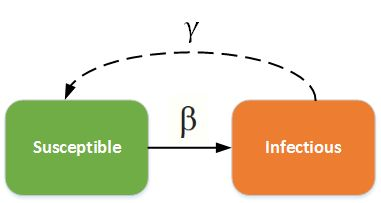为扰动因子#!/usr/bin/python
# -*- coding: utf-8 -*-

import scipy.integrate as spi
import numpy as np
import pylab as pl

beta=1.4247
gamma=0.14286
I0=1e-6
ND=70
TS=1.0
INPUT = (1.0-I0, I0)

def diff_eqs(INP,t):
'''The main set of equations'''
Y=np.zeros((2))
V = INP
Y = - beta * V * V + gamma * V
Y = beta * V * V - gamma * V
return Y   # For odeint

t_start = 0.0; t_end = ND; t_inc = TS
t_range = np.arange(t_start, t_end+t_inc, t_inc)
RES = spi.odeint(diff_eqs,INPUT,t_range)

print(RES)

#Ploting
pl.plot(RES[:,0], '-bs', label='Susceptibles')
pl.plot(RES[:,1], '-ro', label='Infectious')
pl.legend(loc=0)
pl.title('SIS epidemic without births or deaths')
pl.xlabel('Time')
pl.ylabel('Susceptibles and Infectious')
pl.savefig('2.5-SIS-high.png', dpi=900) # This does increase the resolution.
pl.show()
View Code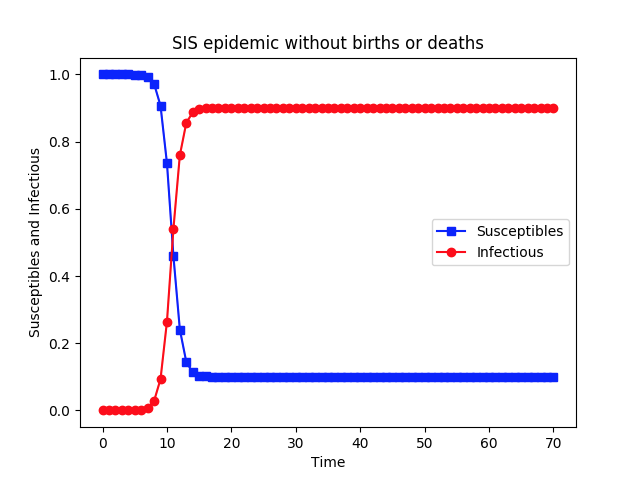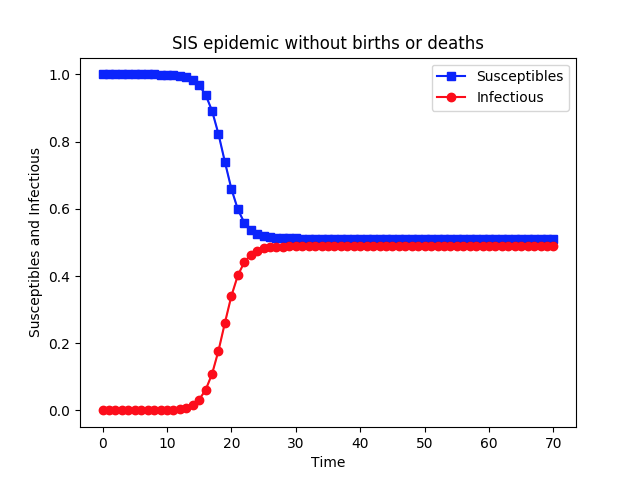gamma=0.7286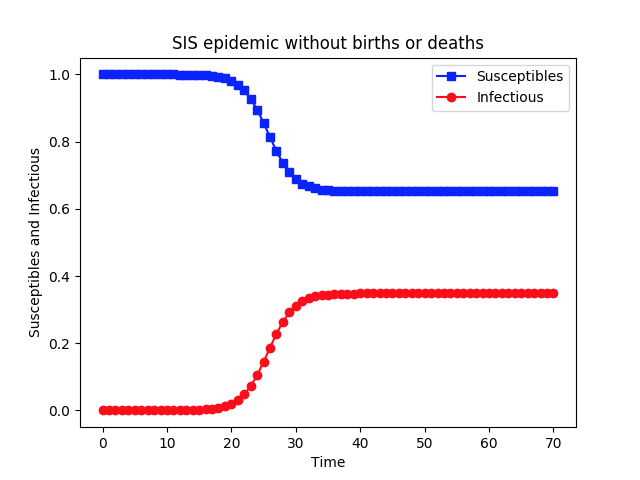gamma=0.9286

### 3. SIR: 急性传染病#!/usr/bin/python
# -*- coding: utf-8 -*-

import scipy.integrate as spi
import numpy as np
import pylab as pl

beta = 1.4247
gamma = 0.14286
TS = 1.0
ND = 70.0
S0 = 1 - 1e-6
I0 = 1e-6
INPUT = (S0, I0, 0.0)

def diff_eqs(INP, t):
'''The main set of equations'''
Y = np.zeros((3))
V = INP
Y = - beta * V * V
Y = beta * V * V - gamma * V
Y = gamma * V
return Y  # For odeint

t_start = 0.0;
t_end = ND;
t_inc = TS
t_range = np.arange(t_start, t_end + t_inc, t_inc)
RES = spi.odeint(diff_eqs, INPUT, t_range)

print(RES)

# Ploting
pl.plot(RES[:, 0], '-bs', label='Susceptibles')  # I change -g to g--  # RES[:,0], '-g',
pl.plot(RES[:, 2], '-g^', label='Recovereds')  # RES[:,2], '-k',
pl.plot(RES[:, 1], '-ro', label='Infectious')
pl.legend(loc=0)
pl.title('SIR epidemic without births or deaths')
pl.xlabel('Time')
pl.ylabel('Susceptibles, Recovereds, and Infectious')
pl.savefig('2.1-SIR-high.png', dpi=900)  # This does, too
pl.show()
View Code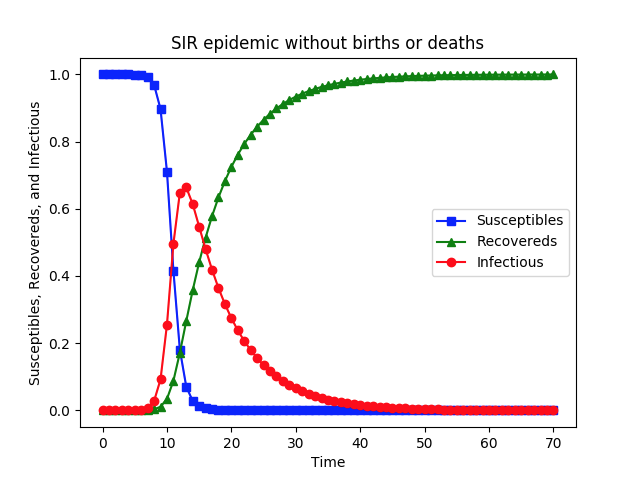### 4. SEIR: 带潜伏期的恶性传染病https://www.meltingasphalt.com/interactive/going-critical/
https://www.bilibili.com/video/av85508117
https://bbs.tiexue.net/post_2895033_1.html
https://blog.csdn.net/wdays83892469/article/details/80878862
https://github.com/zwdnet/2019-nCov-SIRmodel
https://github.com/zwdnet/2019-nCov-SIRmodel/blob/master/data.csv
https://blog.csdn.net/qq_26822029/article/details/104106679
https://www.cnblogs.com/scikit-learn/p/6937326.html
https://github.com/zwdnet/2019-nCov-SIRmodel
https://www.zhihu.com/question/367466399

0
0 收藏

### 作者的其它热门文章0 评论
0 收藏
0